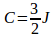# Important Data Interpretation Set – 100

D.1-5) In the following graph, students passing in five years 2008 to 2012 from two schools A and B are given. On the basic of this graph, answer the following questions.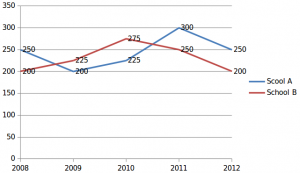1).What is the total number of students passed from both schools during 2009 to 2011?

a) 1525

b) 1475

c) 1425

d) 1375

e) None of these

b) 1475

The total number of students passed from school B during 2009 to 2011 = 225+275+250=750

The total number of students passed from school A during 2009 to 2011 = 200+225+300=725

Total = 750+725=1475

2). The ratio of students passed from school A to those from school B in the years 2010 and 2012 together is

a) 20:19

b) 19:20

c) 5:4

d) 4:5

e) None of these

e) None of these

A Students: B students

= 225+250 : 275+200

= 475:475 = 1:1

3).The total number of students passing from school A from the year 2008 to 2010 is what percentage of the number of students passing from school B from the year 2010 to 2012?

a) 25%

b) 51.35%

c) 74%

d) 91%

e) None of these

e) None of these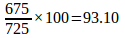4).The average number of students passed from school A and School B?

a) 245 and 230

b) 251 and 231

c) 225 and 255

d) 245 and 212

e) None of these

a) 245 and 230

The average of students passed from school A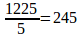The average of students passed from school B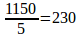5) If the total number of students from school A is 500 each year, the total number of students passed from school A except 2010 is what percent of the total number of students from school A in whole years?

a) 55%

b) 60%

c) 75%

d) 40%

e) None of these

d) 40%

The total number of students from school A in whole years = 2500

The total number of students passed from school A except 2010 = 1000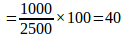D.6-10) The Following data table shows sale of five different motor vehicles across the years. Study it and answer the questions based on it.

 Year Vehicle 2007 2008 2009 2010 2011 Jeep 36 44 49 82 87 Car 27 37 81 37 66 Motorcycle 81 29 75 80 69 Three-wheeler 123 82 107 87 84 Truck 32 49 45 13 37

6) In which of the following two years, the sale of all vehicles taken together is equal?

a) 2007, 2010

b) 2008, 2009

c) 2010, 2011

d) 2007, 2011

e) 2007, 2011

a ) 2007, 2010

Sale of 2007 = sale of 2010 = 299

7) Find the maximum difference of all the vehicles sold over the years.

a) 81

b) 105

c) 116

d) 67

e) 82

c) 116

Total in 2007= 299

2008=241

2009=357

2010=299

2011=343

Maximum difference=357-241=116

8) Trucks sold in 2007 and 2008 taken together is what percent of the trucks sold in the remaining years? (approximately)

a) 80%

b) 83%

c) 85%

d) 84%

e) 89%

c) 85%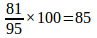9) If three-wheelers sold were rejected due to some fault in the engine in the year 2009, then, total numbers of remaining type of vehicle is approximately what percent of sale of all vehicles in that year?

a) 70%

b) 65%

c) 110%

d) 88%

e)None of these

a) 70%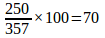10) If the cost of two cars is equal to that of three jeeps. Then one has to purchase nearly how many Jeeps so that their cost is equal to the total cost of cars sold in the year 2010?

a) 55

b) 57

c) 58

d) 69

e) 70